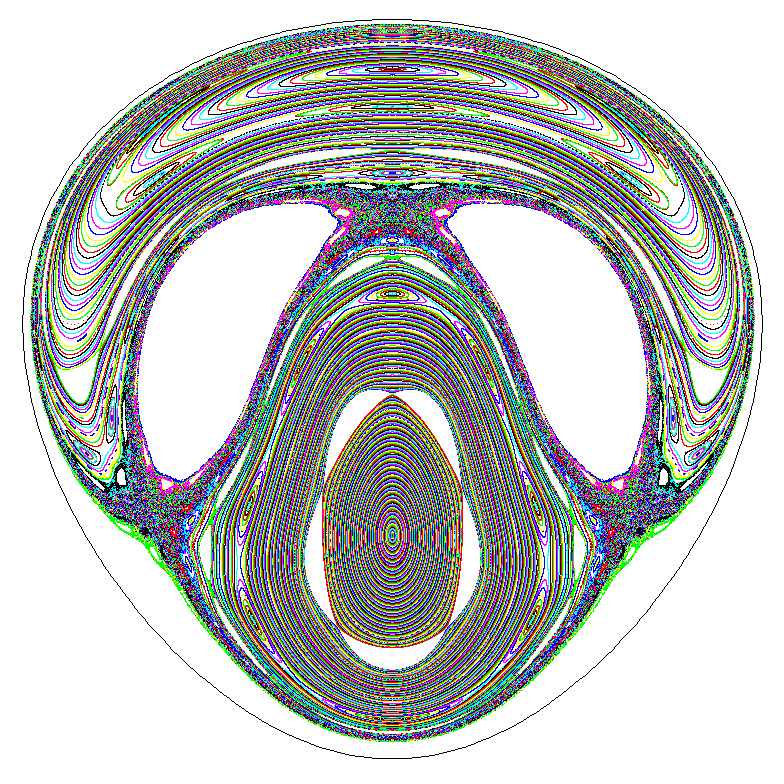The figure displays a Poincaré section of the Hénon-Heiles Hamiltonian,

H=(1/2)(x12+x22+ y12+y22)+ (1/3)x13-x1x22,
using x2=0 as surface of section, and (x1,y1) as coordinates. In the figure the x1 positive axis points downwards and the y1 one to the right. As initial points we have taken 400 points on y1=0 equally spaced between the two elliptic points (on that line) which correspond to elliptic periodic orbits. Note that also the boundary of the section is a periodic orbit. For each one of the initial points 5,000 iterates of the Poincaré map are computed and plotted. In all 2,000,000 Poincaré iterates have been computed. In all these iterates the maximal error in the conservation of the energy is 1.31E-16. Estimated total number of arithmetic operations: 90.65E9. Total (manual cronometer) time: 45 seconds.